Autumn Spice
September 27, 2017

# Autumn Spice

VIEW ALL PHOTOS VIEW SLIDESHOW
GET THE HOW-TO• Formula A

1 ½ oz Joico Vero K-PAK Color 7V + ½ oz Vero K-PAK Color 5RM + 20-volume Vero K-PAK Developer

• Formula B

Add 1 oz Vero K-PAK Color 7XR + 1 oz 30-volume Vero K-PAK Developer to the leftover color from Formula A

• Formula C

Vero K-PAK Chrome V7 + Vero K-PAK Chrome Activator

• Formula D

Vero K-PAK Chrome RR + Vero K-PAK Chrome Activator

• Formula E

Blonde Life Powder Lightener + 20-volume Vero K-PAK Developer# Autumn Spice

Autumn calls for spicy, rich Chambord hues, and this technique from Joico Global Artistic Team Member Gion Vincent definitely delivers. He applies two shades globally in a color melt technique, then adds highlights and lowlights at the same time before finishing with an all-over gloss. The result is insanely shiny, healthy, dimensional hair! Check it out.

Manufacturer: Joico, @joico

## COLOR FORMULAS• Formula A

1 ½ oz Joico Vero K-PAK Color 7V + ½ oz Vero K-PAK Color 5RM + 20-volume Vero K-PAK Developer

• Formula B

Add 1 oz Vero K-PAK Color 7XR + 1 oz 30-volume Vero K-PAK Developer to the leftover color from Formula A

• Formula C

Vero K-PAK Chrome V7 + Vero K-PAK Chrome Activator

• Formula D

Vero K-PAK Chrome RR + Vero K-PAK Chrome Activator

• Formula E

Blonde Life Powder Lightener + 20-volume Vero K-PAK Developer

## HOW-TO STEPS•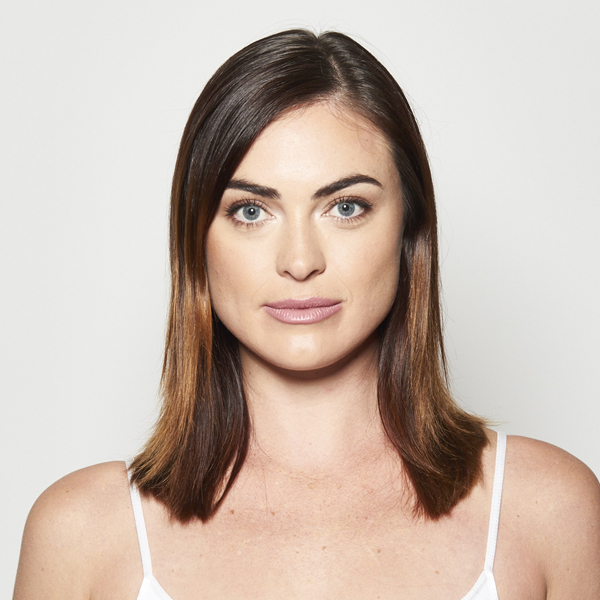• Formula A

1 ½ oz Joico Vero K-PAK Color 7V + ½ oz Vero K-PAK Color 5RM + 20-volume Vero K-PAK Developer

• Formula B

Add 1 oz Vero K-PAK Color 7XR + 1 oz 30-volume Vero K-PAK Developer to the leftover color from Formula A

• Formula C

Vero K-PAK Chrome V7 + Vero K-PAK Chrome Activator

• Formula D

Vero K-PAK Chrome RR + Vero K-PAK Chrome Activator

• Formula E

Blonde Life Powder Lightener + 20-volume Vero K-PAK Developer

1

Before image. The model got a textured, inverted bob by Joico Guest Artist Mandy Kinn, who used a razor for body and movement.

•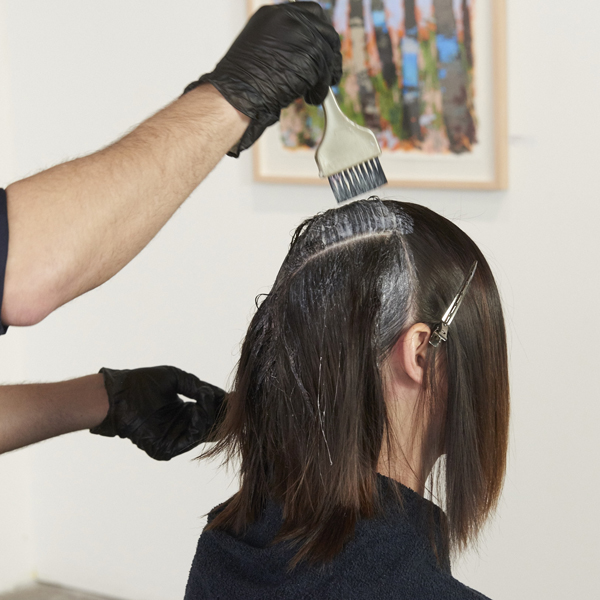• Formula A

1 ½ oz Joico Vero K-PAK Color 7V + ½ oz Vero K-PAK Color 5RM + 20-volume Vero K-PAK Developer

• Formula B

Add 1 oz Vero K-PAK Color 7XR + 1 oz 30-volume Vero K-PAK Developer to the leftover color from Formula A

• Formula C

Vero K-PAK Chrome V7 + Vero K-PAK Chrome Activator

• Formula D

Vero K-PAK Chrome RR + Vero K-PAK Chrome Activator

• Formula E

Blonde Life Powder Lightener + 20-volume Vero K-PAK Developer

2

Start with the hair in an off-center part and apply Formula A in a global application. Bring the color down 2 to 4 inches, depending on the length of the hair. Take 1-inch sections to ensure even saturation.

•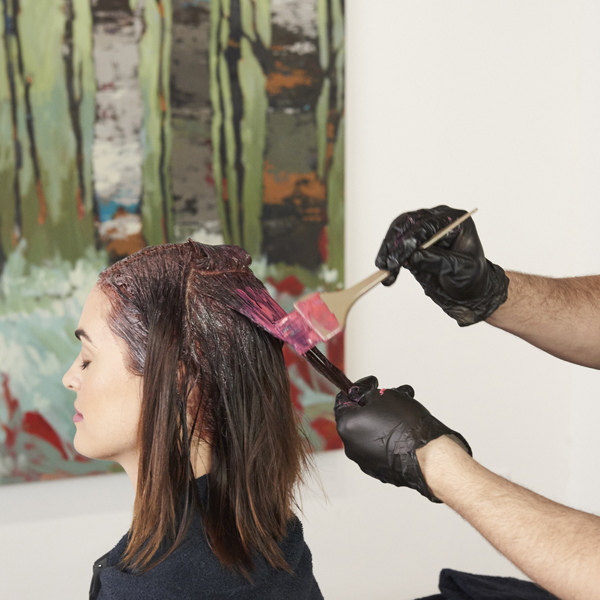• Formula A

1 ½ oz Joico Vero K-PAK Color 7V + ½ oz Vero K-PAK Color 5RM + 20-volume Vero K-PAK Developer

• Formula B

Add 1 oz Vero K-PAK Color 7XR + 1 oz 30-volume Vero K-PAK Developer to the leftover color from Formula A

• Formula C

Vero K-PAK Chrome V7 + Vero K-PAK Chrome Activator

• Formula D

Vero K-PAK Chrome RR + Vero K-PAK Chrome Activator

• Formula E

Blonde Life Powder Lightener + 20-volume Vero K-PAK Developer

3

Add Formula B to the leftover color from Formula A and apply it globally from mids to ends, starting in the back. Use your fingers to melt the the colors together.

•• Formula A

1 ½ oz Joico Vero K-PAK Color 7V + ½ oz Vero K-PAK Color 5RM + 20-volume Vero K-PAK Developer

• Formula B

Add 1 oz Vero K-PAK Color 7XR + 1 oz 30-volume Vero K-PAK Developer to the leftover color from Formula A

• Formula C

Vero K-PAK Chrome V7 + Vero K-PAK Chrome Activator

• Formula D

Vero K-PAK Chrome RR + Vero K-PAK Chrome Activator

• Formula E

Blonde Life Powder Lightener + 20-volume Vero K-PAK Developer

4

Process for 30 minutes, then rinse and blow-dry.

•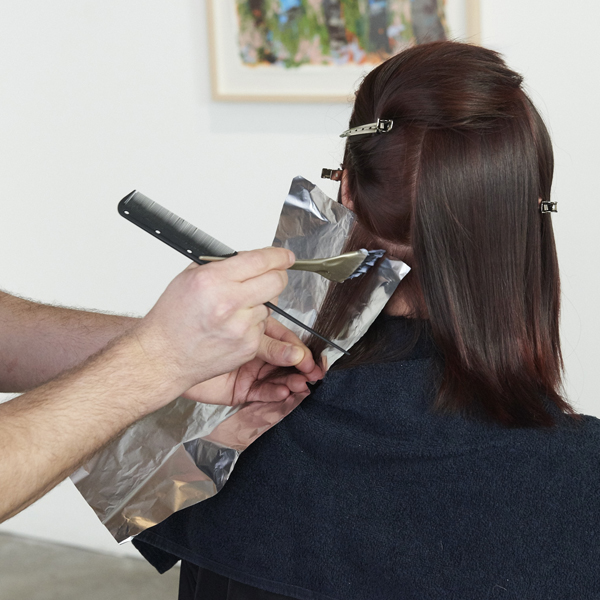• Formula A

1 ½ oz Joico Vero K-PAK Color 7V + ½ oz Vero K-PAK Color 5RM + 20-volume Vero K-PAK Developer

• Formula B

Add 1 oz Vero K-PAK Color 7XR + 1 oz 30-volume Vero K-PAK Developer to the leftover color from Formula A

• Formula C

Vero K-PAK Chrome V7 + Vero K-PAK Chrome Activator

• Formula D

Vero K-PAK Chrome RR + Vero K-PAK Chrome Activator

• Formula E

Blonde Life Powder Lightener + 20-volume Vero K-PAK Developer

5

Part the hair into quadrants. Take a diagonal slice at the nape, then take a fine slice from the top of this first slice. Lay the fine slice on a foil and apply Formula C in a triangle shape on the foil.

•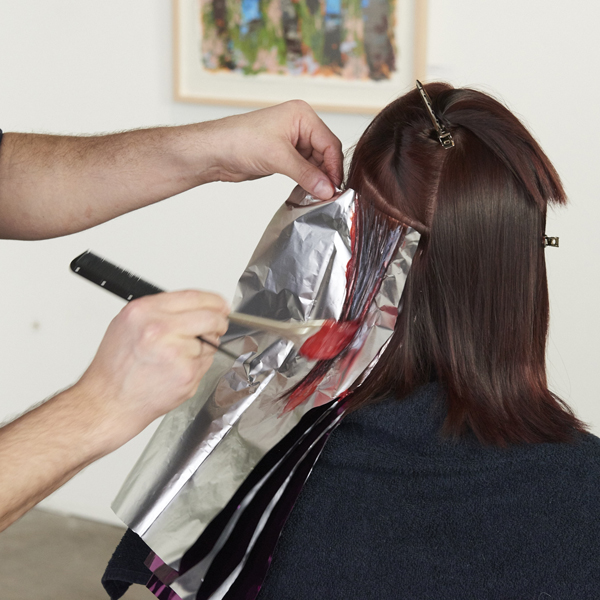• Formula A

1 ½ oz Joico Vero K-PAK Color 7V + ½ oz Vero K-PAK Color 5RM + 20-volume Vero K-PAK Developer

• Formula B

Add 1 oz Vero K-PAK Color 7XR + 1 oz 30-volume Vero K-PAK Developer to the leftover color from Formula A

• Formula C

Vero K-PAK Chrome V7 + Vero K-PAK Chrome Activator

• Formula D

Vero K-PAK Chrome RR + Vero K-PAK Chrome Activator

• Formula E

Blonde Life Powder Lightener + 20-volume Vero K-PAK Developer

6

On the same foil, apply Formula D in a V-shape on the rest of the hair through the ends, surrounding the triangle of Formula C. These will be your lowlights. Continue for six slices.

•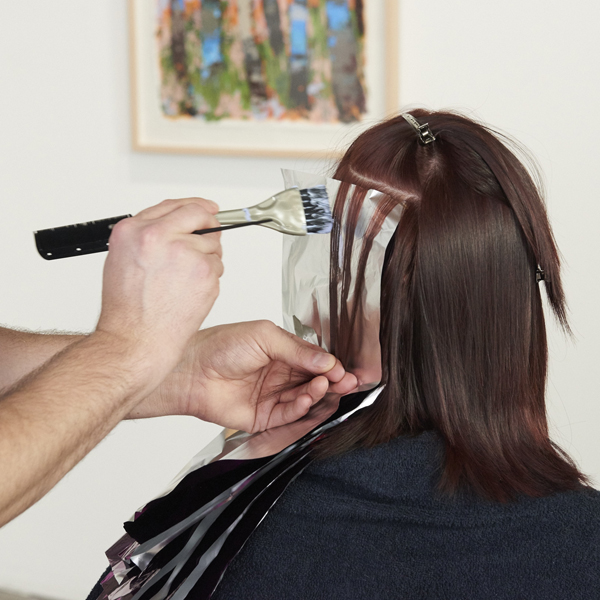• Formula A

1 ½ oz Joico Vero K-PAK Color 7V + ½ oz Vero K-PAK Color 5RM + 20-volume Vero K-PAK Developer

• Formula B

Add 1 oz Vero K-PAK Color 7XR + 1 oz 30-volume Vero K-PAK Developer to the leftover color from Formula A

• Formula C

Vero K-PAK Chrome V7 + Vero K-PAK Chrome Activator

• Formula D

Vero K-PAK Chrome RR + Vero K-PAK Chrome Activator

• Formula E

Blonde Life Powder Lightener + 20-volume Vero K-PAK Developer

7

After six slices, take a weave slice and apply Formula E. This will be your highlight. Take another slice above the highlight and apply Formulas C and D in the lowlight technique, alternating between highlights and lowlights up the head. Repeat on the other side.

•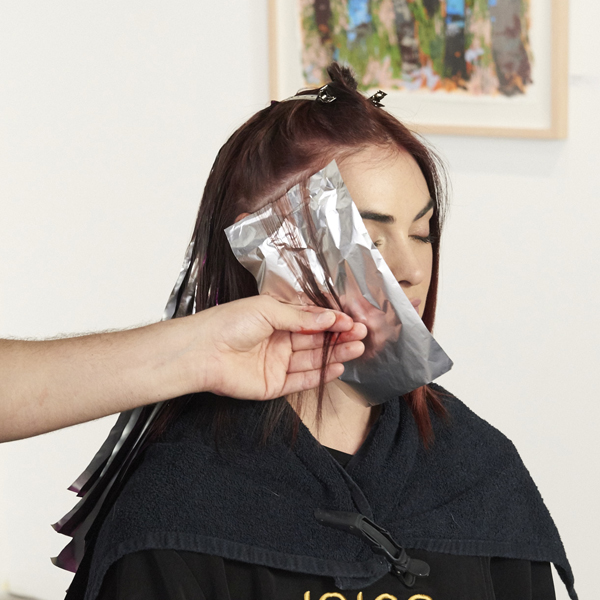• Formula A

1 ½ oz Joico Vero K-PAK Color 7V + ½ oz Vero K-PAK Color 5RM + 20-volume Vero K-PAK Developer

• Formula B

Add 1 oz Vero K-PAK Color 7XR + 1 oz 30-volume Vero K-PAK Developer to the leftover color from Formula A

• Formula C

Vero K-PAK Chrome V7 + Vero K-PAK Chrome Activator

• Formula D

Vero K-PAK Chrome RR + Vero K-PAK Chrome Activator

• Formula E

Blonde Life Powder Lightener + 20-volume Vero K-PAK Developer

8

On the front sections, start with a diagonal slice at the hairline. Weave out a section and apply the highlight with Formula E. Take another slice behind that, weave and apply Formula E. Behind the second highlight, take a slice, then a fine slice off the top of that, and apply Formulas C and D as the lowlight.

•• Formula A

1 ½ oz Joico Vero K-PAK Color 7V + ½ oz Vero K-PAK Color 5RM + 20-volume Vero K-PAK Developer

• Formula B

Add 1 oz Vero K-PAK Color 7XR + 1 oz 30-volume Vero K-PAK Developer to the leftover color from Formula A

• Formula C

Vero K-PAK Chrome V7 + Vero K-PAK Chrome Activator

• Formula D

Vero K-PAK Chrome RR + Vero K-PAK Chrome Activator

• Formula E

Blonde Life Powder Lightener + 20-volume Vero K-PAK Developer

9

Alternate up the head, then repeat on the other side. Process for 15 minutes or until highlights reach the desired lightness, then rinse and blow-dry.

•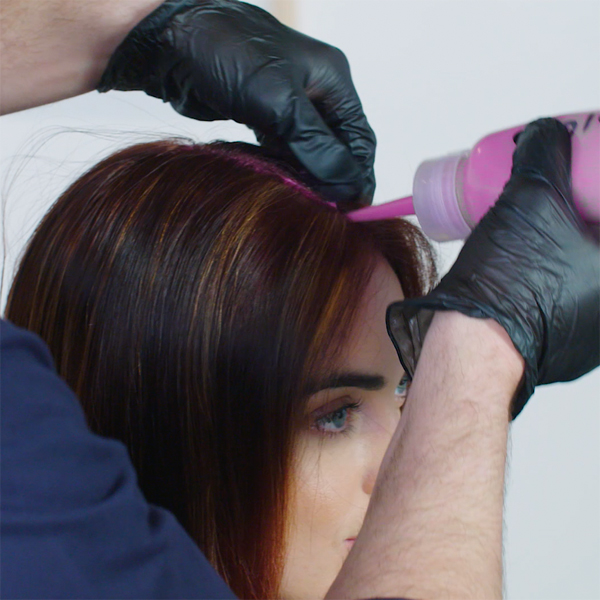• Formula A

1 ½ oz Joico Vero K-PAK Color 7V + ½ oz Vero K-PAK Color 5RM + 20-volume Vero K-PAK Developer

• Formula B

Add 1 oz Vero K-PAK Color 7XR + 1 oz 30-volume Vero K-PAK Developer to the leftover color from Formula A

• Formula C

Vero K-PAK Chrome V7 + Vero K-PAK Chrome Activator

• Formula D

Vero K-PAK Chrome RR + Vero K-PAK Chrome Activator

• Formula E

Blonde Life Powder Lightener + 20-volume Vero K-PAK Developer

10

Apply Formula F globally using a bottle. Gion stresses that applying the gloss on dry hair is crucial, as the formula will absorb better. Process for 10 minutes, then shampoo with Joico K-PAK Color Therapy. Style as desired.

•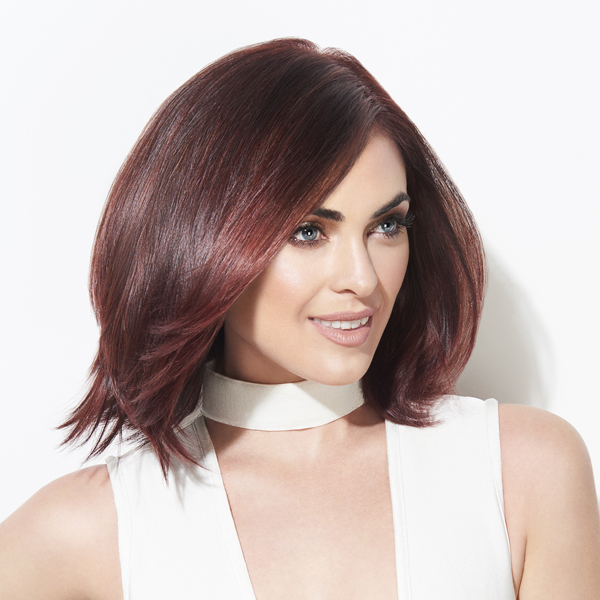• Formula A

1 ½ oz Joico Vero K-PAK Color 7V + ½ oz Vero K-PAK Color 5RM + 20-volume Vero K-PAK Developer

• Formula B

Add 1 oz Vero K-PAK Color 7XR + 1 oz 30-volume Vero K-PAK Developer to the leftover color from Formula A

• Formula C

Vero K-PAK Chrome V7 + Vero K-PAK Chrome Activator

• Formula D

Vero K-PAK Chrome RR + Vero K-PAK Chrome Activator

• Formula E

Blonde Life Powder Lightener + 20-volume Vero K-PAK Developer

11

Finished look.